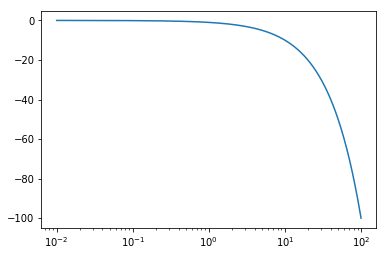# 45. Asymptotic Bode diagrams¶

One of the big advantages of Bode diagrams is that they are very easy to sketch out by hand (or, equivalently, to visualise mentally).

:

import numpy
import matplotlib.pyplot as plt
%matplotlib inline

:

omega = numpy.logspace(-2, 2, 1000)
s = 1j*omega


# 46. Systems with real poles¶

Let’s study the bode diagrams of systems of the form

$\frac{K}{(\tau s + 1)^n}$
:

def annotated_bode(ax_gain, ax_phase, G, K, tau, order):
high_freq_asymptote = K/(tau*omega)**order

# Gain part
ax_gain.loglog(omega, numpy.abs(G))
ax_gain.axhline(K, color='grey')  # Rule 1
ax_gain.loglog(omega, high_freq_asymptote, color='grey') # Rule 2
ax_gain.axvline(1/tau, color='grey')  # Rule 2
ax_gain.set_ylim([1e-2, 1e+1])
ax_gain.set_ylabel('|G|')

# Phase part
ax_phase.axhline(0, color='grey')  # Rule 3
ax_phase.semilogx(omega, numpy.unwrap(numpy.angle(G)))
ax_phase.axhline(-numpy.pi/2*order, color='grey')  # Rule 4
ax_phase.axvline(1/tau, color='grey')  # Rule 5
ax_phase.set_ylim([-3*numpy.pi/2, 2*numpy.pi/2])
ax_phase.set_ylabel(r'$\angle G$')

:

def plotresponse(order=1, tau=1, K=1):
G = K/(tau*s + 1)**order

fig, [ax_gain, ax_phase] = plt.subplots(2, 1)
annotated_bode(ax_gain, ax_phase, G, K, tau, order)

:

from ipywidgets import interact

:

interact(plotresponse, order=(-2, 3), tau=(0.1, 10), K=(-1., 2));


We see that we can construct a reasonable approximation by knowing a couple of things

1. The gain ($$K$$) of the system defines the low frequency asymptote of the gain graph
2. The high frequency asymptote of the gain is $$\frac{K}{(\omega\tau)^n}$$. Effectively, on a loglog scale, this means we have -n/decade slope above frequencies of around $$1/\tau$$
3. The low frequency phase asymptote is 0
4. The high frequency phase asymptote is $$-n\pi/2$$
5. The phase curve has an inflection at $$1/\tau$$

# 47. Systems with complex poles¶

Systems with complex poles show uniique frequency response behaviour. We will focus on the second order system shown below:

$G = \frac{K}{\tau^2 s^2 + 2\tau\zeta s + 1}$
:

def plotresponse(K=1, tau=1, zeta=1):
plt.figure(figsize=(15, 5))
order = 2
G = K/(tau**2*s**2 + 2*tau*zeta*s + 1)

ax_gain = plt.subplot2grid((2, 2), (0, 0))
ax_phase = plt.subplot2grid((2, 2), (1, 0))
ax_complex = plt.subplot2grid((2, 2), (0, 1), rowspan=2)

annotated_bode(ax_gain, ax_phase, G, K, tau, order)

# poles
poles = numpy.roots([tau**2, 2*tau*zeta, 1])
ax_complex.scatter(poles.real, poles.imag)
ax_complex.axhline(0)
ax_complex.axvline(0)
ax_complex.axis([-2, 2, -2, 2])

:

interact(plotresponse, K=(0.1, 2), tau=(0.1, 2), zeta=(0., 1.1))

:

<function __main__.plotresponse(K=1, tau=1, zeta=1)>


We see that the rules from before still hold, except that we start seeing the so-called “harmonic nose” emerge when $$\zeta<\sqrt{2}/2\approx{0.7}$$. The maximum of the nose occurs at the resonant frequency of

$\omega_r = \frac{\sqrt{1 - 2\zeta^2}}{\tau}$

The effect of dead time is to increase the phase lag indefinitely as a function of frequency. Delay has no effect on the gain of a system.

:

D = 1
G = numpy.exp(-D*s)

:

plt.semilogx(omega, numpy.unwrap(numpy.angle(G)))

:

[<matplotlib.lines.Line2D at 0x117a944e0>]